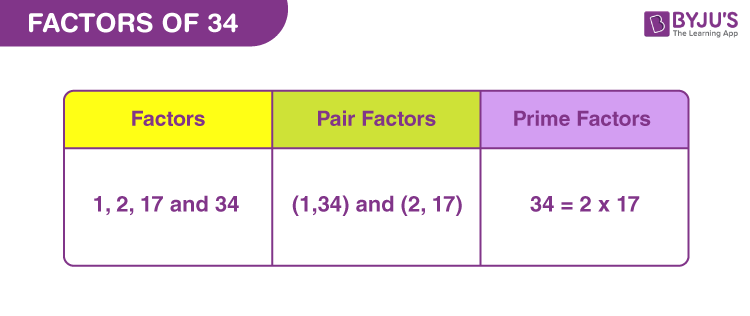# Factors of 34

Factors of 34 are the integers that divide the original number evenly and the resulting quotient is a whole number. Since 34 is a composite number, it will have more than two factors.

Multiples and factors of 34 are different from each other. Multiples are divisible by the original number whereas factors of a number divide the original number. But 34 is both a factor and multiple of itself.

Now let us learn to find the factors of 34 along with pair factors and prime factors.## How to Find Factors of 34?

Factors of 34 are the numbers that can divide the original number. Now to find the factors, we need to divide the original number by natural numbers, till the quotient is 1. Firstly, we will divide 34 by the smallest natural number, 1.

• 34 ÷ 1 = 34
• 34 ÷ 2 = 17
• 34 ÷ 17 = 2
• 34 ÷ 34 = 1

Thus, we can conclude that only 1, 2, 17 and 34 are the factors of 34.

## Pair Factors of 34

To find the pair factors of 34, we need to find the product of the two numbers, to get the original number.

• 1 × 34 = 34
• 2 × 17 = 34

Hence, the pair factors are (1, 34) and (2, 17).

The negative pair factors of 34 will also result in the original number, after multiplication. Therefore,

• -1 × -34 = 34
• -2 × -17 = 34

Therefore, the negative pair factors are (-1, -34) and (-2, -17).

## Prime Factorisation of 34

Prime factors are the prime numbers that have only two factors. The examples are 2, 3, 5, 7, 11, etc. Now to find the prime factors of 34, we need to check if 34 is completely divisible by that prime number or not. Thus, we will start dividing 34 by the smallest prime factor, i.e.,2.

Step 1: Divide 34 by the smallest prime factor.

34/2 = 17

Step 2: Since 17 itself is a prime number, therefore, it is divisible by 17 only.

17/17 = 1

Step 3: Now further division is not possible. Thus, we will consider 2 and 7 as the prime factors of 34.

 Prime factorisation of 34 = 2 x 17

## Solved Examples

Q.1: Meera is distributing candies in her class on her birthday. If there are 34 candies and each student gets 2 candies, then how many students are present in a class?

Solution: Given,

Number of candies = 34

Number of candies each student gets = 2

Therefore, number of students = 34/2 = 17

Q.2: Find the sum of all the factors of 34.

Solution: The factors of 34 are 1, 2, 17 and 34.

Sum = 1+2+17+34 = 54

Therefore, 54 is the required sum.

Q.3: What are the common factors of 33 and 34?

Answer: Both 33 and 34 are composite numbers. Thus, their factors are:

33 → 1, 3, 11 and 33

34 → 1, 2, 17 and 34

Therefore, the common factor of 33 and 34 is only 1.

## Frequently Asked Questions on Factors of 34

### 1. How many factors of 34 are there?

Answer: There are four factors of 34. They are 1, 2, 17 and 34.

### 2. Is 34 a prime number or a composite number?

Answer: 34 is not a prime number but a composite number, since it has more than two factors.

### 3. What two factors make 34?

Answer: When we multiply 2 by 17, we get 34. Thus, 2 and 17 are two such factors, whose product gives the original number.

### 4. What is the largest prime factor of 34?

Answer: The largest prime factor of 34 is 17.

### 5. What are the multiples of 34?

Answer: The first 10 multiples of 34 are:

34, 68, 102, 136, 170, 204, 238, 272, 306 and 340

### 6. What are the prime factors of 34?

Answer: The prime factorisation of 34 is equal to 2 x 17. Therefore, 2 and 17 are the prime factors of 34.